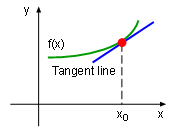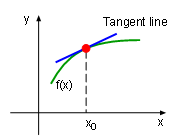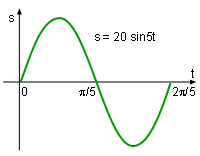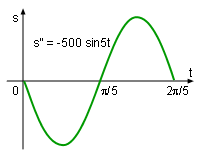Ch 4. The Mean Value Theorem Multimedia Engineering Math Maximum & Minimum Rolle'sTheorem Mean ValueTheorem MonotonicFunctions First DerivativeTest Concavity &Inflection SecondDerivative Test
 Chapter 1. Limits 2. Derivatives I 3. Derivatives II 4. Mean Value 5. Curve Sketching 6. Integrals 7. Inverse Functions 8. Integration Tech. 9. Integrate App. 10. Parametric Eqs. 11. Polar Coord. 12. Series Appendix Basic Math Units Search eBooks Dynamics Fluids Math Mechanics Statics Thermodynamics Author(s): Hengzhong Wen Chean Chin Ngo Meirong Huang Kurt Gramoll ©Kurt GramollMATHEMATICS - THEORY In this section, the Test for Concavity is introduced along with an application. ConcavityIncreasing Function IIncreasing Function II The concept of increasing and decreasing function has been introduced in the Monotonic Function section. However, an increasing function can have two different bend directions as shown in Increasing Function I and Increasing Function II graphic respectively. In order to distinguish the two kinds of bend direction, the concept of concave upward and cave downward is introduced. An arc is concave upward if the arc lays above the tangent line for every point in the function's domain. For example, the curve in Increasing Function I is concave upward. Similarly, an arc is concave downward if the arc lays below the tangent line for every point in the function's domain. For example, the curve in Increasing Function II is concave downward.Function s = 20 sin5t In engineering, data curves and relationships are complex and in general composed of both concave upward and concave downward. In the Higher Order Derivatives section, the displacement and time relationship of a spring-mounted slider was introduced as s = 20 sin5t. According to the concavity definition, the curve is concave downwards from 0 to π/5, but concave upward form π/5 to 2π/5. To help find the concavity of a curve, the Test for Concavity can be used. The Test for Concavity The test for concavity states: Suppose a function, f(x), is twice differentiable on an interval R. If the second derivative of the function, df2(x)/dx2 , is larger than 0 for all x in R, then the graph of the function is concave upward on R. If the second derivative of the function, df2(x)/dx2 , is less than 0 for all x in R, then the graph of the function is concave downward on RFunction d2s/dt2 = -500 sin5t The test for concavity can be better understood by examining the displacement and time relationship of a spring-mounted slider. First, assume the displacement over time is given by s = 20 sin5t. The first derivative of the displacement function with respect to time is      ds/dt = 100 cos5t The second derivative of the displacement function with respect to time is      d2s/dt2 = -500 sin5t This expression is negative when t is between 0 and π/5. According to the test of concavity it is concave downward in this range. On the other hand, This expression is positive when t is between π/5 and 2π/5, and thus it is concave upward in this interval. This result is confirmed by Function s = 20 sin5t graphic. Inflection Points The curve of spring-mounted slider function changes from concave downward to concave upward when t = π/5. In engineering this point is known as an inflection point. The inflection point is the point where the curve changes from concave upward to concave downward or from concave downward to concave upward.